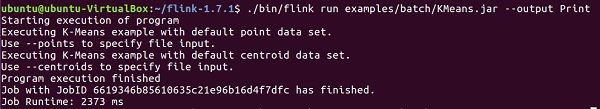# Apache Flink - Machine Learning

Apache Flink's Machine Learning library is called FlinkML. Since usage of machine learning has been increasing exponentially over the last 5 years, Flink community decided to add this machine learning APO also in its ecosystem. The list of contributors and algorithms are increasing in FlinkML. This API is not a part of binary distribution yet.

Here is an example of linear regression using FlinkML −

```// LabeledVector is a feature vector with a label (class or real value)
val trainingData: DataSet[LabeledVector] = ...
val testingData: DataSet[Vector] = ...

// Alternatively, a Splitter is used to break up a DataSet into training and testing data.
val dataSet: DataSet[LabeledVector] = ...
val trainTestData: DataSet[TrainTestDataSet] = Splitter.trainTestSplit(dataSet)
val trainingData: DataSet[LabeledVector] = trainTestData.training
val testingData: DataSet[Vector] = trainTestData.testing.map(lv => lv.vector)
val mlr = MultipleLinearRegression()

.setStepsize(1.0)
.setIterations(100)
.setConvergenceThreshold(0.001)
mlr.fit(trainingData)

// The fitted model can now be used to make predictions
val predictions: DataSet[LabeledVector] = mlr.predict(testingData)
```

Inside flink-1.7.1/examples/batch/ path, you will find KMeans.jar file. Let us run this sample FlinkML example.

This example program is run using the default point and the centroid data set.

```./bin/flink run examples/batch/KMeans.jar --output Print
```